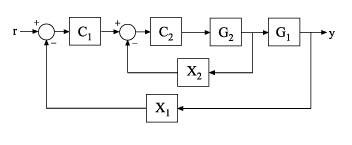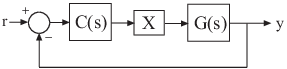# getLoopTransfer

Open-loop transfer function of control system

## Syntax

``L = getLoopTransfer(T,Locations)``
``L = getLoopTransfer(T,Locations,sign)``
``L = getLoopTransfer(T,Locations,sign,openings)``

## Description

example

````L = getLoopTransfer(T,Locations)` returns the point-to-point open-loop transfer function of a control system measured at specified analysis points. The point-to-point open-loop transfer function is the open-loop response obtained by injecting signals at the specified locations and measuring the return signals at the same locations. ```

example

````L = getLoopTransfer(T,Locations,sign)` specifies the feedback sign for calculating the open-loop response. The relationship between the closed-loop response `T` and the open-loop response `L` is `T = feedback(L,1,sign)`.```

example

````L = getLoopTransfer(T,Locations,sign,openings)` specifies additional loop-opening locations to open for computing the open-loop response at `Locations`. ```

## Examples

collapse all

Compute the open-loop response of the following control system model at an analysis point specified by an `AnalysisPoint` block, `X`.Create a model of the system by specifying and connecting a numeric LTI plant model, `G`, a tunable controller, `C`, and the `AnalysisPoint`, `X`.

```G = tf([1 2],[1 0.2 10]); C = tunablePID('C','pi'); X = AnalysisPoint('X'); T = feedback(G*X*C,1);```

`T` is a `genss` model that represents the closed-loop response of the control system from $r$ to $y$. The model contains `AnalysisPoint` block `X`, which identifies the potential loop-opening location.

Calculate the open-loop point-to-point loop transfer at location `X`.

`L = getLoopTransfer(T,'X');`

This command computes the positive-feedback transfer function you would obtain by opening the loop at `X`, injecting a signal into `G`, and measuring the resulting response at the output of `C`. By default, `getLoopTransfer` computes the positive feedback transfer function. In this example, the positive feedback transfer function is $L\left(s\right)=-G\left(s\right)C\left(s\right)$

The output `L` is a `genss` model that includes the tunable block `C`. You can use `getValue` to obtain the current value of `L`, in which all the tunable blocks of `L` are evaluated to their current numeric value.

Compute the negative-feedback open-loop transfer of the following control system model at an analysis point specified by an `AnalysisPoint` block, `X`.Create a model of the system by specifying and connecting a numeric LTI plant model `G`, a tunable controller `C`, and the `AnalysisPoint` block `X`.

```G = tf([1 2],[1 0.2 10]); C = tunablePID('C','pi'); X = AnalysisPoint('X'); T = feedback(G*X*C,1);```

`T` is a `genss` model that represents the closed-loop response of the control system from $r$ to $y$. The model contains the `AnalysisPoint` block `X` that identifies the potential loop-opening location.

Calculate the open-loop point-to-point loop transfer at the location `X`.

`L = getLoopTransfer(T,'X',-1);`

This command computes the open-loop transfer function from the input of `G` to the output of `C`, assuming that the loop is closed with negative feedback. That is, the relationships between `L` and `T` is given by `T = feedback(L,1)`. In this example, the positive feedback transfer function is $L\left(s\right)=G\left(s\right)C\left(s\right)$

Compute the open-loop response of the inner loop of the following cascaded control system, with the outer loop open.Create a model of the system by specifying and connecting the numeric plant models `G1` and `G2`, the tunable controllers `C1`, and the `AnalysisPoint` blocks `X1` and `X2` that mark potential loop-opening locations.

```G1 = tf(10,[1 10]); G2 = tf([1 2],[1 0.2 10]); C1 = tunablePID('C','pi'); C2 = tunableGain('G',1); X1 = AnalysisPoint('X1'); X2 = AnalysisPoint('X2'); T = feedback(G1*feedback(G2*C2,X2)*C1,X1);```

Compute the negative-feedback open-loop response of the inner loop, at the location `X2`, with the outer loop opened at `X1`.

`L = getLoopTransfer(T,'X2',-1,'X1');`

By default, the loop is closed at the analysis-point location marked by the `AnalysisPoint` block `X1`. Specifying `'X1'` for the `openings` argument causes `getLoopTransfer` to open the loop at `X1` for the purposes of computing the requested loop transfer at `X2`. In this example, the negative-feedback open-loop response $L\left(s\right)={G}_{2}\left(s\right){C}_{2}\left(s\right)$.

## Input Arguments

collapse all

Model of a control system, specified as a Generalized State-Space (`genss`) Model. Locations at which you can open loops and perform open-loop analysis are marked by `AnalysisPoint` blocks in `T`.

Analysis-point locations in the control system model at which to compute the open-loop point-to-point response, specified as a character vector or a cell array of character vectors that identify analysis-point locations in `T`.

Analysis-point locations are marked by `AnalysisPoint` blocks in `T`. An `AnalysisPoint` block can have single or multiple channels. The `Location` property of an `AnalysisPoint` block gives names to these feedback channels.

The name of any channel in an `AnalysisPoint` block in `T` is a valid entry for the `Locations` argument to `getLoopTransfer`. To get a full list of available analysis points in `T`, use `getPoints(T)`.

`getLoopTransfer` computes the open-loop response you would obtain by injecting a signal at the implicit input associated with an `AnalysisPoint` channel, and measuring the response at the implicit output associated with the channel. These implicit inputs and outputs are arranged as follows.`L` is the open-loop transfer function from `in` to `out`.

Feedback sign, specified as `+1` or `-1` The feedback sign determines the sign of the open-loop transfer function.

• `+1` — Compute the positive-feedback loop transfer. In this case, the relationship between the closed-loop response `T` and the open-loop response `L` is ```T = feedback(L,1,+1)```.

• `-1` — Compute the negative-feedback loop transfer. In this case, the relationship between the closed-loop response `T` and the open-loop response `L` is ```T = feedback(L,1)```.

Choose a feedback sign that is consistent with the conventions of the analysis you intend to perform with the loop transfer function. For example, consider the following system, where `T` is the closed-loop transfer function from r to y.To compute the stability margins of this system with the `margin` command, which assumes negative feedback, you need to use the negative-feedback open-loop response. Therefore, you can use `L = getLoopTransfer(T,'X',-1)` to obtain the negative-feedback transfer function L = GC.

Additional locations for opening feedback loops for computation of the open-loop response, specified as character vector or cell array of character vectors that identify analysis-point locations in `T`. Analysis-point locations are marked by `AnalysisPoint` blocks in `T`. Any channel name contained in the `Location` property of an `AnalysisPoint` block in `T` is a valid entry for `openings`.

Use `openings` when you want to compute the open-loop response at one analysis-point location with other loops also open at other locations. For example, in a cascaded loop configuration, you can calculate the inner loop open-loop response with the outer loop also open. Use `getPoints(T)` to get a full list of available analysis-point locations in `T`.

## Output Arguments

collapse all

Point-to-point open-loop response of the control system `T` measured at the analysis points specified by `Locations`, returned as a generalized state-space (`genss`) model.

• If `Locations` specifies a single analysis point, then `L` is a SISO `genss` model. In this case, `L` represents the response obtained by opening the loop at `Locations`, injecting signals and measuring the return signals at the same location.

• If `Locations` is a vector signal, or specifies multiple analysis points, then `L` is a MIMO `genss` model. In this case, `L` represents the open-loop MIMO response obtained by opening loops at all locations listed in `Locations`, injecting signals and measuring the return signals at those locations.

## Tips

• You can use `getLoopTransfer` to extract open-loop responses given a generalized model of the overall control system. This is useful, for example, for validating open-loop responses of a control system that you tune with the a tuning command such as `systune`.

• `getLoopTransfer` is the `genss` equivalent to the Simulink® Control Design™ command `getLoopTransfer`, which works with the `slTuner` and `slLinearizer` interfaces. Use the Simulink Control Design command when your control system is modeled in Simulink.